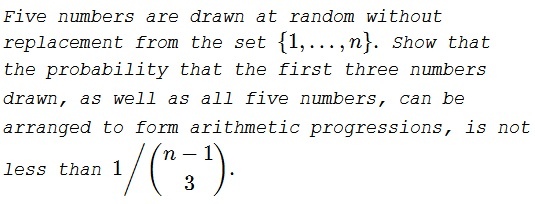# Random Arithmetic Progressions

### Problem### Solution 1

There are $\displaystyle {n\choose 5}$ ways to choose $4$ numbers out of $n.$ This is the size of our sample space. Not all five-term sequences admit an arrangement into an arithmetic progression. Those that do, can be identified by two numbers, say, $x$ and $y,$ playing the role of the smallest and the largest terms of the progression. $x$ and $y$ need to satisfy $x\equiv y\text{ (mod }4).$ Let $N(n)$ be the number of such pairs. Thus the probability of getting a five-term arithmetic progression equals $\displaystyle P_5=\frac{N(n)}{{n\choose 5}}.$

If five numbers $\{a_0,a_1,a_2,a_3,a_4\}$ form an arithmetic progression then so do $\{a_0,a_1,a_2\},$ $\{a_1,a_2,a_3\},$ $\{a_2,a_3,a_4\},$ and $\{a_1,a_3,a_5\}.$ In other words, out of $\displaystyle 10={5\choose 3}$ possible selections of three elements, only four amount to an arithmetic progression. Combining the two estimates in one, the probability in question equals

$\displaystyle P_{5,3}=\fracN(n)\cdot\frac{4}{10}}{n\choose 5}}$

If $n=4m,$ $\displaystyle N(4m) = 4\cdot\frac{m(m-1)}{2}=\frac{n(n-4)}{8},$ because there are $4$ remainders modulo $4$ and, for each, $m$ terms that can be paired with other $m-1$ terms.

Now if $n=4m-k,$ where $k=1,2,3,$ define $n'=n+k.$ As before, there are $\displaystyle \frac{n'(n'-4)}{8}$ pairs of numbers but several have been just added. So to correct the mishap,

\displaystyle \begin{align} N(n)&=\frac{n'(n'-4)}{8}-k\left(\frac{n'}{4}-1\right)\\ &=\frac{(n+k)(n+k-4)-2k(n+k-4)}{8}\\ &=\frac{(n+k-4)(n-4)}{8}=\frac{n^2-4n}{8}+\frac{4k-k^2}{8}\\ &\ge\frac{n(n-4)}{8} \end{align}

because $k\lt 4.$ Thus, in any event $\displaystyle N(n)\ge\frac{n(n-4)}{8},$ implying

\displaystyle\begin{align}P_{5,3}&=\fracN(n)\cdot\frac{4}{10}}{n\choose 5}}\\ &\ge\frac{4\cdot 12\cdot n(n-4)}{8\cdot n(n-1)(n-2)(n-3)(n-4)}\\ &=\frac{6}{(n-1)(n-2)(n-3)}=\frac{1}{{n-1\choose 3}}. \end{align

### Solution 2

$\textbf{Denominator}$ There are $\displaystyle \frac{n!}{(n-5)!}$ permutations of subsample $S=\{1,\ldots,n\}$.

$\textbf{Numerator}$ We look for $\xi=\left\lfloor \frac{n}{4}\right\rfloor$.

$\textbf{General solution for 5}$

We have arithmetic sequences (5 members) separated by $\zeta=\{1, 2,\ldots, \xi\}$, such that the sequence will be expressed as $a_1, a_1+\zeta, a_1+2 \zeta \ldots a_1+4\zeta$, and constrained by $a_1+4 \zeta\leq n$.

We can show that there are $n-4 \zeta$ possible increasing sequences of progression of difference $\zeta$

There are $5!$ permutations for any 5 selected numbers. The number of sequences

$\displaystyle \sum _{\zeta=1}^{\xi } (n-4 \zeta)=-2 \xi ^2+n \xi -2 \xi$

The probability of having a progression becomes

$\displaystyle p=\frac{5! \left(-2 \xi ^2+n \xi -2 \xi \right)}{\frac{n!}{(n-5)!}}$

For large $n$ or values of $n$ divisible by 4,

$\displaystyle p=\frac{15}{(n-3) (n-2) (n-1)},$

which is greater than $\displaystyle \frac{1}{\binom{n-1}{3}}=\frac{6}{(n-3) (n-2) (n-1)}$.

$\textbf{General solution for 3 then 5}$

Conditional on having the draws with arithmetic sequence $\{X_{(1)},X_{(2)},\ldots,X_{(5)}\}$ in various permutations of $\frac{5!}{(5-3)!}=60$ counts of first three draws, how many include a sequence?

We have 6 counts for $\{X_{(1)},X_{(2)},X_{(3)}\}$, that is $3!$, plus 6 counts of $\{X_{(2)},X_{(3)},X_{(4)}\}$ , plus 6 counts of $\{X_{(3)},X_{(4)},X_{(5)}\}$, plus 6 counts of plus 6 counts of $\{X_{(1)},X_{(3)},X_{(5)}\}$, for a total of 24 counts so the correction is

$\displaystyle p \times \frac{24}{60}$.

### Acknowledgment

This is a slight modification of a problem proposed by Iceland for the 1987 IMO that was discussed in R. Honsberger's From Erdös To Kiev (MAA, 1996, 37-40).

Second solution is by N. N. Taleb.